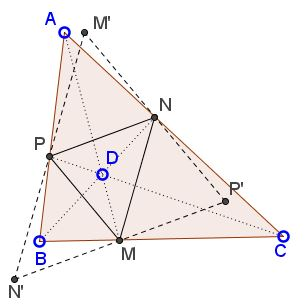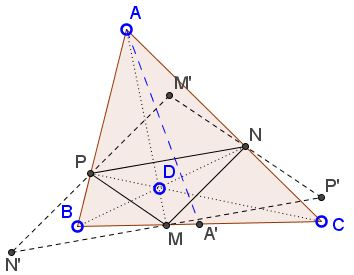# Cevian Parallelogram

### Problem

Given $\Delta ABC$ and point $D$ within the triangle. Let $M,$ $N,$ $P,$ be the feet of the cevians $AM,$ $BN,$ $CP$ through point $D.$Then there exists point $S$ within $\Delta ABC$ such that the four points $M,$ $N,$ $P,$ and $S$ form a parallelogram.

### Proof

Construct $\Delta M'N'P'$ for which $\Delta MNP$ serves the medial triangle.There are three parallelograms with vertices $M,$ $N,$ $P:$ $MNPN',$ $NPMP',$ and $PMNM'.$ Thus the problem will be solved if we prove that one of the points $M',$ $N',$ $P',$ lies within $\Delta ABC.$

Note that if, say, $D$ lies on the median $AA',$ i.e., if $M=A',$ then $NP\parallel BC$ and both $N'$ and $P'$ lie on the side line $BC.$ Denote this side line $\alpha.$Except for the case $D\in AA',$ points $N'$ and $P'$ lie on different sides of line $\alpha.$ Clearly, $N'$ and $P'$ cannot lie on the same side of $\alpha$ because $N'P'$ crosses $\alpha$ at $M.$ Moreover, one of $N'$ or $P'$ that lies on the same side of $AA'$ as $D,$ lies on the side of $\alpha$ opposite $A.$ (In the diagram below this is $N'),$ while $P'$ lies on the same side of $\alpha$ as $A.$This is because sliding $M$ away from $A'$ towards, say, $B$ pushes $P$ down $AB$ and $N$ up $AC.$ Relative to $\alpha$ the movement of $N'$ and $P'$ is opposite to that of $N$ and $P,$ respectively.

In general, the endpoints of the segments $M'N',$ $N'P',$ and $P'M'$ are on different sides of relevant side lines and cross in and out of $\Delta ABC$ at points $P,$ $M,$ and $N,$ respectively. We can see that all three points $M',$ $N',$ $P'$ cannot be outside $\Delta ABC.$Indeed, assume that, say, $N'$ and $P'$ are outside. Then lines $N'M'$ and $P'M'$ cross at $P$ and $N$ from outside inside, implying that they also meet (at point $M')$ inside $\Delta ABC$ ($\Delta APN,$ actually.)

Note: An absolutely analogous behavior is observed when $A,$ $N,$ $P$ are the pedal points of $D.$ This is discussed on a separate page.